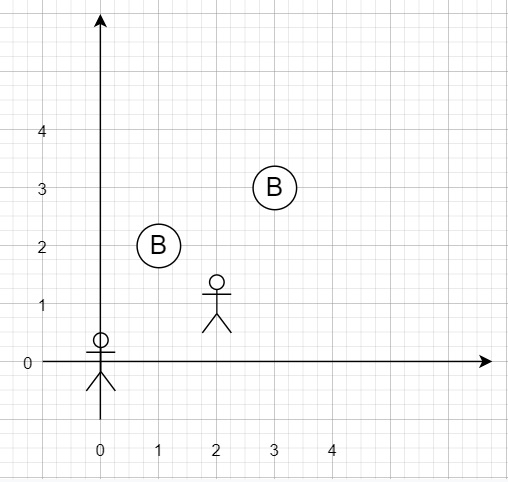# Campus Bikes II in Python

Suppose we have a 2D grid, that represents a campus, there are N workers and M bikes, The value of N <= M. Now Each worker and bike are in a 2D coordinate on this grid. So, if we want to assign one unique bike to each worker so that the sum of the Manhattan distances between each worker and their assigned bike is minimum.

We know that the Manhattan distance between two points p1 and p2 is (p1, p2) = |p1.x - p2.x| + |p1.y - p2.y|. We have to find the minimum possible sum of Manhattan distances between each worker and their assigned bike.

So, if the input is like workers = [[0,0],[2,1]], bikes = [[1,2],[3,3]]then the output will be 6

To solve this, we will follow these steps −

• Define a function helper(). This will take a,b

• return |a-b| + |a - b|

• Define a function solve(). This will take bikes, workers,bikev,i:= 0

• info := a list with i and bikev

• if info is present in memo, then

• return memo[info]

• if i is same as size of workers, then

• return 0

• temp := infinity

• for j in range 0 to size of bikes, do

• if not bikev[j] is non-zero, then

• bikev[j]:= 1

• temp := minimum of temp, helper(workers[i], bikes[j]) +solve(bikes, workers, bikev, i+1)

• bikev[j]:= 0

• memo[info]:= temp

• return temp

• Define a function assignBikes(). This will take workers, bikes

• bikev := a list whose size is same as the size of bikes, fill this with false

• memo:= a new map

• return solve(bikes, workers, bikev)

## Example

Let us see the following implementation to get a better understanding −

Live Demo

class Solution(object):
def helper(self,a,b):
return abs( (a-b) ) + abs( (a - b) )
def solve(self,bikes,workers,bikev,i=0):
info = (i,tuple(bikev))
if info in self.memo:
return self.memo[info]
if i == len(workers):
return 0
temp = float('inf')
for j in range(len(bikes)):
if not bikev[j]:
bikev[j]=1
temp = min(temp,self.helper(workers[i],bikes[j])+self.solve(bikes,workers,bi
kev,i+1))
bikev[j]=0
self.memo[info]= temp
return temp
def assignBikes(self, workers, bikes):
bikev = [False for i in range(len(bikes))]
self.memo={}
return self.solve(bikes,workers,bikev)
ob = Solution()
print(ob.assignBikes([[0,0],[2,1]],[[1,2],[3,3]]))

## Input

[[0,0],[2,1]] [[1,2],[3,3]]

## Output

6